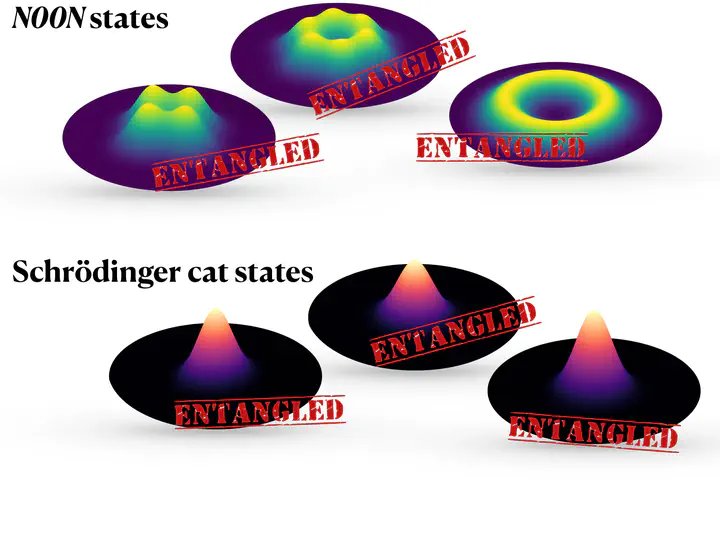# Entropic entanglement now published in PRA

Our latest work has appeared in Physical Review A. We derive a new set of entanglement criteria in phase space that uses the Husimi distribution.Example phase space distributions in the twisted coordinates. These are some of the example states we consider in our work.

Our new paper, Entropic entanglement criteria in phase space, has recently been published in Physical Review A!

It is well known that entanglement is incredibly hard not just to measure, but also to witness. In our new paper, we develop a new method to witness entanglement that relies on measuring the entropy.

Entropy in information theory measures how much missing information or ‘uncertainty’ there is with a particular measurement. A common example used to conceptualise entropy is a biased coin flip. Say we have a coin that has probability $p$ of landing on heads, and probability $1-p$ to land on tails. If $p=0.5$, the entropy is maximised. This is because before we make the measurement, there is maximal uncertainty to what the result will be. Now, if $p=0$ or $p=1$, the entropy is zero! We already know what the outcome will be before even flipping the coin, and hence there is no uncertainty in the measurement of the coin.

Entropy is a promising resource for measuring entanglement as it captures the entire probability distribution of a particular measurement. Many entanglement measures rely on second-order moments of a distribution, but entropies can capture all the moments. Entropic entanglement measures already exist, however our method exploits a different type of measurement that measures a particular distribution known as the Husimi distribution, $Q(x,p)$. The Husimi distribution has an entropy associated to it called the Wehrl entropy, $S_W(Q)$.

The Husimi distribution contains all the information of the quantum state, and the great thing about it is that it can be measured! In quantum optics systems, it is well-established that the Husimi distribution is accessed via heterodying (see, e.g., this book by Schleich). More recently, it has been shown the Husimi distribution can be measured in spinor Bose-Einstein condensates (see this recent preprint).

In our paper, we consider two subsystems $1$ and $2$. They have canonical variables $x_j$ and $p_j$ that satisfy the commutation relation $[x_j,p_j] = i\hbar\delta_{jk}$. Jumping straight to the result, our method relies on measuring the Wehrl entropy of the two local subsystems $S_W(Q_1)$ and $S_W(Q_2)$, as well as measuring the entropy of a system with ’twisted coordinates’. This system uses both subsystems $1$ and $2$ to create new variables $x_\pm = x_1\pm x_2$ and $p_\mp = p_1 \mp p_2$ that defines a distribution $Q_\pm(x_\pm,p_\mp)$. We then measure the entropy of this distribution, $S_M(Q_\pm)$ (we use subscript $M$ here as it is technically no longer a true Wehrl entropy).

Our entanglement witness is then defined as

$$S_M(Q_\pm) \geq \ln\left(e^{S_W(Q_1)} + e^{S_W(Q_2)}\right).$$

These are known as the strong criteria and apply to pure states. We can similarly define a weak criteria by invoking some properties of the Wehrl entropy. The weak criteria is thus

$$S_M(Q_\pm) \geq 1 + \ln2,$$

describing both pure and mixed states. A violation of these bounds flag entanglement. In our paper, we consider some examples of different quantum states to show our new entanglement witness works well. We consider both Gaussian and non-Gaussian states and show that our witness is stronger than previous witnesses for certain non-Gaussian states, such as the Schrödinger cat state.

An infographic that describes the main points of our preprint can be found here.